20.1
19.2
19.1
18.2
18.1
17.2
The page you are viewing does not exist in version 17.2. This link will take you to the root page.

# Whole and Visual Ranges

Whole and visual axis ranges define which data an axis displays. The whole range is automatically calculated based on the data of all series associated with the axis. A chart does not show values that are outside the range. A visual range is equal to a whole range by default and determines the data range the Chart control currently displays.

An x-axis's visual range is equal to a whole range An x-axis's visual range is less than a whole range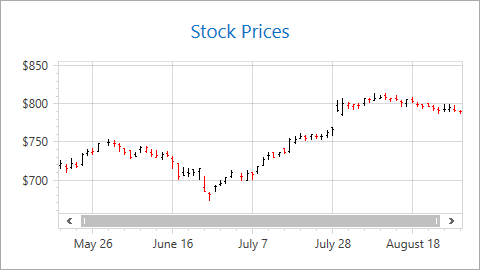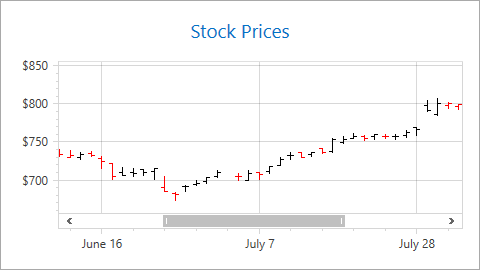##### NOTE

The following axes types allow you to operate with their whole or visual ranges:

This document consists of the following sections:

## How End User Can Change a Visual Range

An end user changes a visual range when zooming and scrolling a chart. Note that, changing the visual range does not affect the whole range.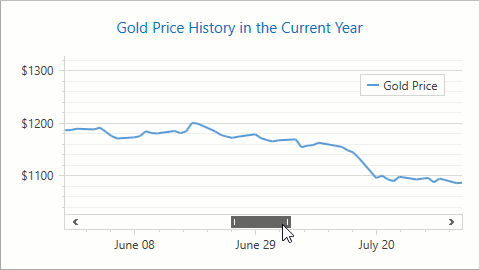Use the markup below to enable scrolling and zooming in a chart:

``````<dxc:XYDiagram2D EnableAxisXNavigation="True"
<!--...-->
</dxc:XYDiagram2D>
``````

The code uses the following classes and properties:

Class or Property Description
XYDiagram2D The XY Diagram.
XYDiagram2D.EnableAxisXNavigation Specifies whether an end user can scroll and zoom a chart's x-axis.
XYDiagram2D.EnableAxisYNavigation Specifies whether an end user can scroll and zoom a chart's y-axis.

## How to Configure Visual and Whole Ranges

The following markup shows how to specify visual and whole ranges:

``````<!--...-->
<dxc:XYDiagram2D.AxisX>
<dxc:AxisX2D>
<dxc:AxisX2D.WholeRange>
<dxc:Range MinValue="05/24/2016"
MaxValue="08/25/2016"/>
</dxc:AxisX2D.WholeRange>
<dxc:AxisX2D.VisualRange>
<dxc:Range MinValue="06/18/2016"
MaxValue="07/03/2016"/>
</dxc:AxisX2D.VisualRange>
</dxc:AxisX2D>
</dxc:XYDiagram2D.AxisX>
<!--...-->
``````

The markup above uses the following classes and properties:

Class or Property Description
Axis.WholeRange Gets or sets the axis whole range's settings.
Axis2D.VisualRange Gets or sets the axis visual range's settings.
Range The axis range.
Range.MinValue The range's minimum limit.
Range.MaxValue The range's maximum limit.

You can call the Range.SetMinMaxValues method to limit a range at runtime. The following code customizes an x-axis's visual range:

``````using DevExpress.Xpf.Charts;

private void Window_Loaded(object sender, RoutedEventArgs e) {
Range visualRange = ((XYDiagram2D)chartControl1.Diagram).ActualAxisX.ActualVisualRange;
visualRange.SetMinMaxValues(minValue: new DateTime(2019, 4, 1), maxValue: new DateTime(2019, 10, 1));
}
``````

## How to Specify Diagram Side Margins

The Chart Control adds additional margins between the outermost series point and the diagram's edge. To configure margins, use the Range.StartSideMargin, Range.EndSideMargin or Range.SideMarginsValue property.

AutoSideMargins = true

SideMarginsValue = 0

(AutoSideMargins = false)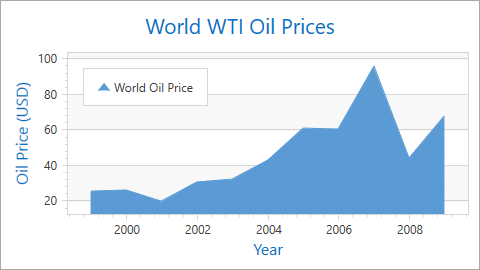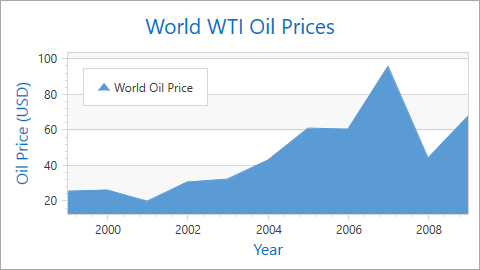The following example shows how to remove margins:

Markup:

``````<dxc:XYDiagram2D.AxisX>
<dxc:AxisX2D x:Name="xAxis">
<dxc:AxisX2D.WholeRange>
<dxc:Range SideMarginsValue="0"/>
</dxc:AxisX2D.WholeRange>
</dxc:AxisX2D>
</dxc:XYDiagram2D.AxisX>
``````

At runtime:

``````Range range = new Range();
xAxis.WholeRange = range;
range.SideMarginsValue=0;
``````

The SideMarginSizeUnit property defines margin measurement units. When SideMarginSizeUnit is set to SideMarginSizeUnit.AxisUnit (the default value), margins are specified in axis measurement units.

To define margins as a percentage of the axis range, set Range.SideMarginSizeUnit to SideMarginSizeUnit.AxisRangePercentage.

The example below demonstrates how to set the left margin as a percentage: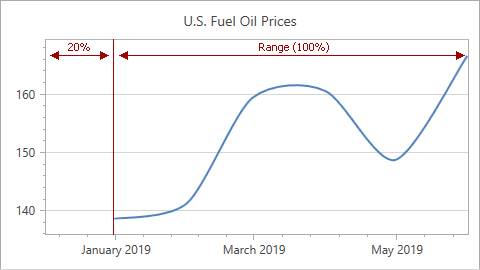Markup:

``````<dxc:ChartControl ... >
<dxc:XYDiagram2D>
<dxc:XYDiagram2D.AxisX>
<dxc:AxisX2D x:Name="xAxis" ...>
<dxc:AxisX2D.WholeRange>
<dxc:Range StartSideMargin="20"
SideMarginSizeUnit="AxisRangePercentage"/>
</dxc:AxisX2D.WholeRange>
</dxc:AxisX2D>
</dxc:XYDiagram2D.AxisX>
</dxc:XYDiagram2D>
...
</dxc:ChartControl>
``````

At runtime:

``````Range range = new Range();
xAxis.WholeRange = range;
range.SideMarginSizeUnit = SideMarginSizeUnit.AxisRangePercentage;
range.StartSideMargin = 20;
``````

If you specify the SideMarginsValue, StartSideMargin or EndSideMargin property, the control ignores the Range.AutoSideMargins property.

## How to Hide Zero Level on a Chart Value Axis

When a y-axis range is automatically calculated, you can use the AlwaysShowZeroLevel property to hide the zero value level. In this case, a new optimal minimum value is specified for the y-axis:

AlwaysShowZeroLevel = true AlwaysShowZeroLevel = false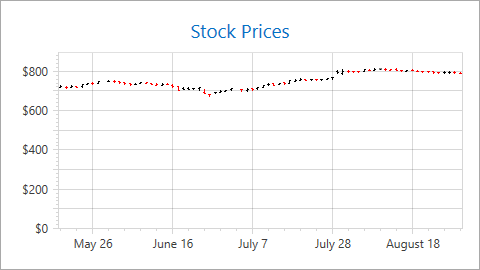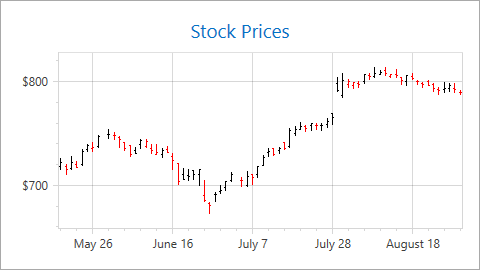Use the code below to hide a y-axis's zero level:

``````<dxc:XYDiagram2D>
<dxc:XYDiagram2D.AxisY>
<dxc:AxisY2D>
<dxc:AxisY2D.WholeRange>
<dxc:Range dxc:AxisY2D.AlwaysShowZeroLevel="False"/>
</dxc:AxisY2D.WholeRange>
</dxc:AxisY2D>
</dxc:XYDiagram2D.AxisY>
<!--...-->
</XYDiagram2D>
``````

The markup uses the following classes and properties:

Class or Property Description
Axis.WholeRange The axis's whole range.
Range An axis's range.
AxisY2D.AlwaysShowZeroLevel Specifies whether to show the XY Diagram's y-axis zero level.

Use the following properties to hide the zero level in 3D charts and circular charts (Polar and Radar series):

## How to Configure Y-Axis's Range Based on X-Axis's Visual Range

The XYDiagram2D.DependentAxesYRange option allows you to only display y-axis values for point values that are in the x-axis's visual range.

DependentAxesYRange = false DependentAxesYRange = true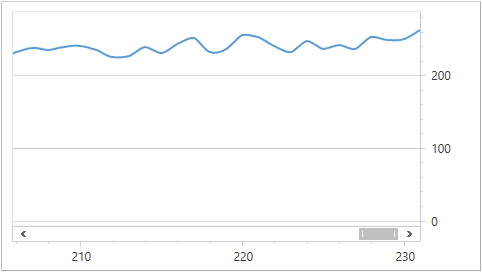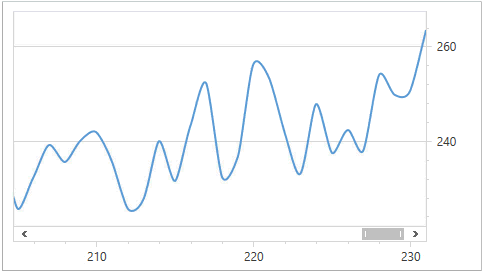The following markup shows how to do this:

``````<dxc:ChartControl>
<dxc:XYDiagram2D DependentAxesYRange="True">
<dxc:XYDiagram2D.AxisX>
<dxc:AxisX2D StickToEdge="True"/>
</dxc:XYDiagram2D.AxisX>
<!--...-->
</dxc:XYDiagram2D>
</dxc:ChartControl>
``````

The markup uses the following classes and properties:

Class or Property Description
XYDiagram2D.DependentAxesYRange Gets or sets the value indicating whether all y-axes' visual ranges should be calculated only by point values in the x-axis's visual range.
AxisX2D.StickToEdge Gets or sets the value that specifies whether the visual range sticks to the whole range's start or end.